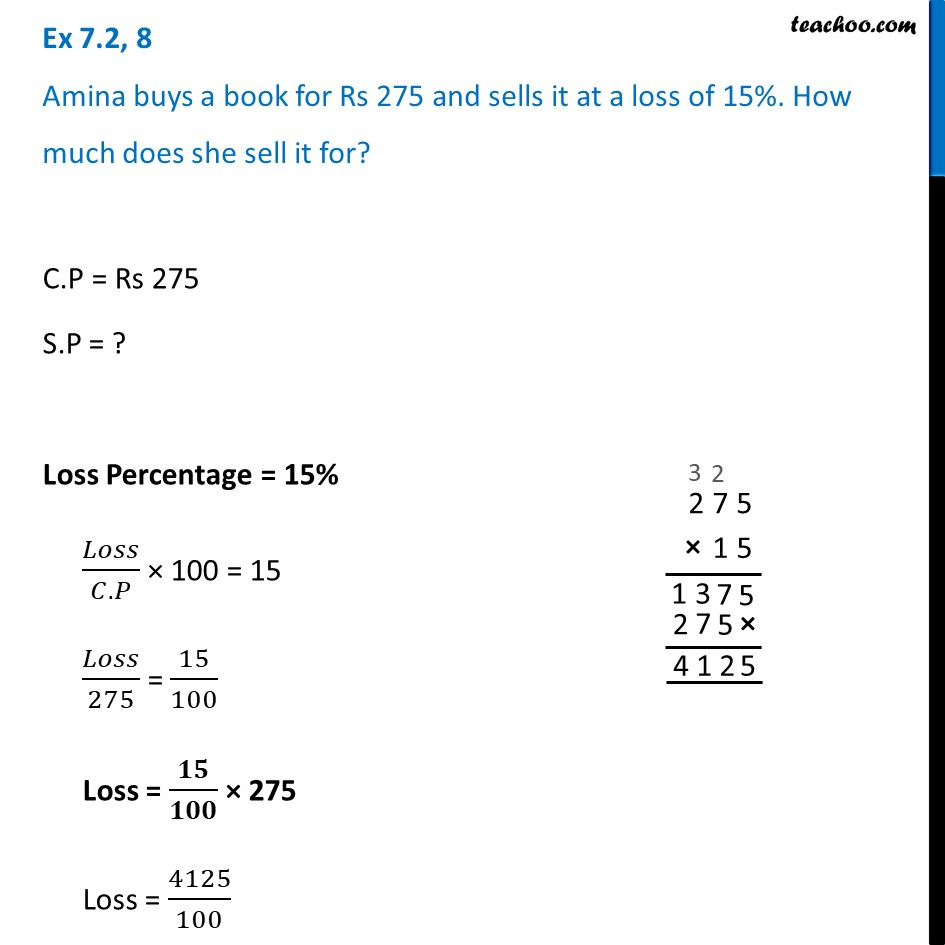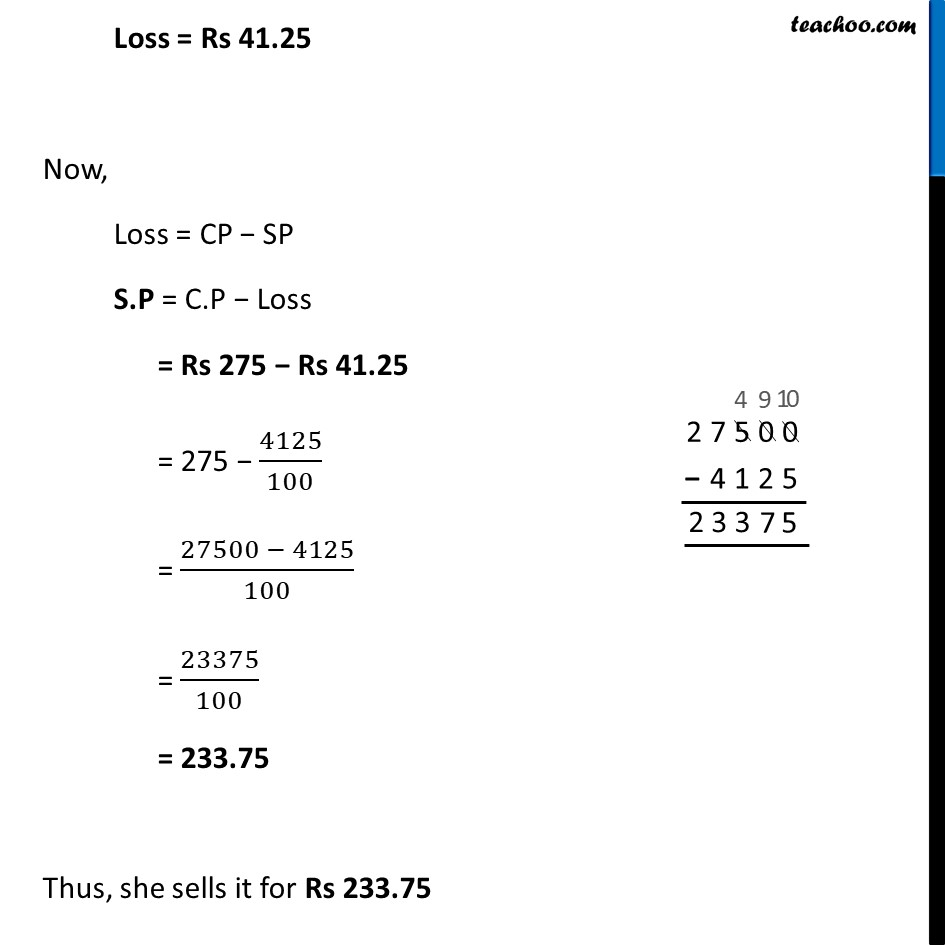Ex 7.2

Chapter 7 Class 7 Comparing Quantities
Serial order wiseLearn in your speed, with individual attention - Teachoo Maths 1-on-1 Class

### Transcript

Ex 7.2, 8 Amina buys a book for Rs 275 and sells it at a loss of 15%. How much does she sell it for? C.P = Rs 275 S.P = ? Loss Percentage = 15% 𝐿𝑜𝑠𝑠/(𝐶.𝑃) × 100 = 15 𝐿𝑜𝑠𝑠/275 = 15/100 Loss = 𝟏𝟓/𝟏𝟎𝟎 × 275 Loss = 4125/100 Loss = Rs 41.25 Now, Loss = CP − SP S.P = C.P − Loss = Rs 275 − Rs 41.25 = 275 − 4125/100 = (27500 − 4125)/100 = 23375/100 = 233.75 Thus, she sells it for Rs 233.75